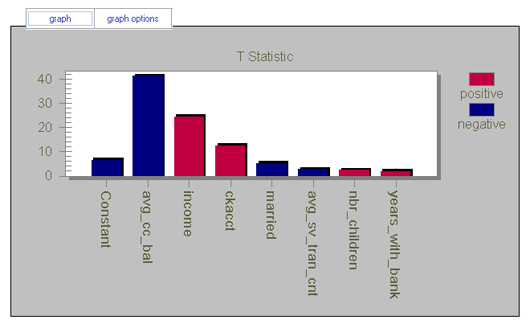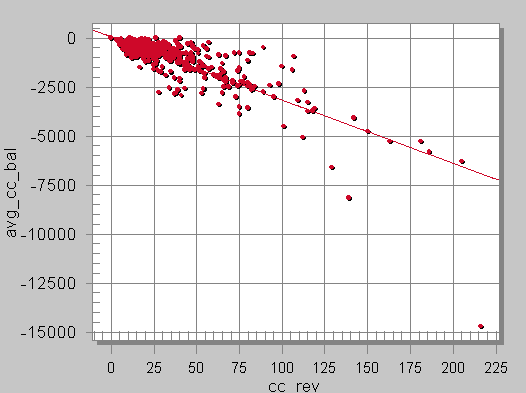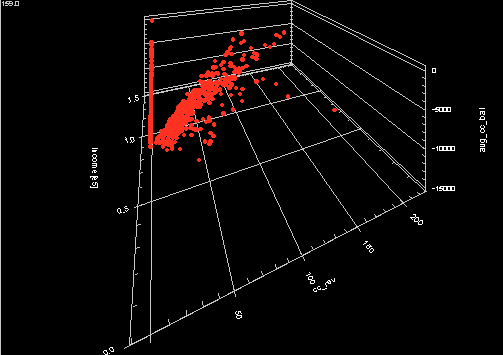# 5.4.5 - Linear Weights Graph - Teradata Warehouse Miner

## Teradata Warehouse Miner User Guide - Volume 3Analytic Functions

Product
Release Number
5.4.5
Published
February 2018
Language
English (United States)
Last Update
2018-05-04
dita:mapPath
yuy1504291362546.ditamap
dita:ditavalPath
ft:empty
By default, the Linear Weights graph displays the relative magnitudes of the T-statistic associated with each coefficient in the linear regression model:
Linear Regression Tutorial: Linear Weights Graph1. Select the Graphics Options tab.
2. Change the Graph Type to Standardized Coefficient to view the standardized coefficient values.

Although not generated automatically, a Scatter Plot is useful for analyzing the model built with the Linear Regression analysis. As an example, a scatter plot is brought up to look at the dependent variable (“cc_rev”), with the first two independent variables that made it into the model (“avg_cc_bal,” “income”). Create a new Scatter Plot analysis, and pick these three variables in the Selected Tables and Columns option. The results are shown first in two dimensions (avg_cc_bal and cc_rev), and then with all three:

Linear Regression Tutorial: Scatter Plot (2d)Linear Regression Tutorial: Scatter Plot (3d)